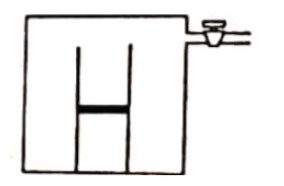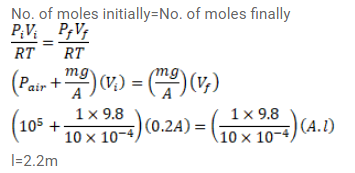# Use R=8.3 J/mol-K wherever required. An ideal gas is kept in a long cylindrical vessel fittedQuestion:

Use $\mathrm{R}=8.3 \mathrm{~J} / \mathrm{mol}-\mathrm{K}$ wherever required.

An ideal gas is kept in a long cylindrical vessel fitted with a frictionless piston of cross-sectional area 10 $\mathrm{cm}^{2}$ and weight $1 \mathrm{~kg}$. The vessel itself is kept in a big chamber containing air at atmospheric pressure $100 \mathrm{kPa}$. The length of the gas column is $20 \mathrm{~cm}$. If the chamber is now completely evacuated by an exhaust pump, what will be the length of the gas column? Assume the temperature to remain constant throughout the process.Solution: